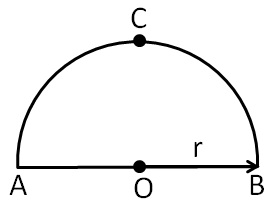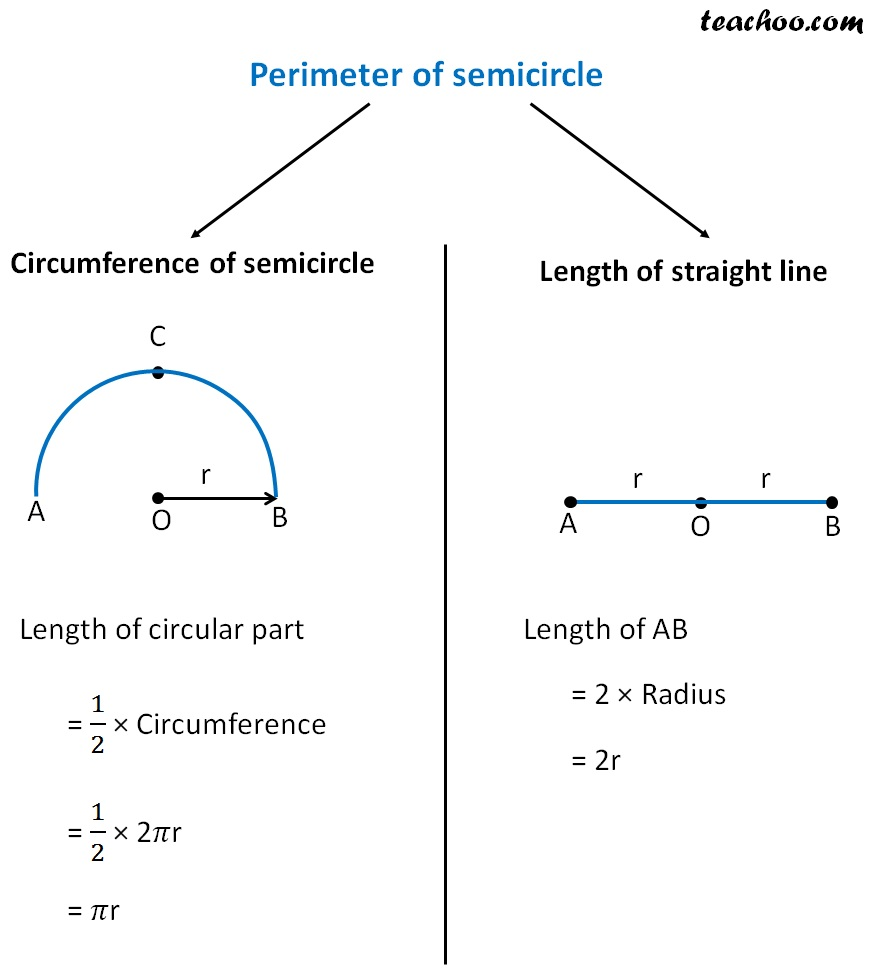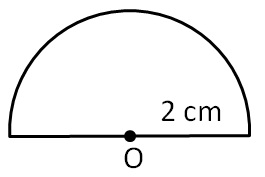Perimeter of a combination of shapes

Chapter 11 Class 7 Perimeter and Area
Concept wise

Let us consider a semicircle ABCPerimeter of semicircle will be the distance around itSo,

Perimeter of semicircle = 𝜋r + 2 r

= r(𝜋 + 2)

Let us take an example:

#### Find perimeter of a semicircle with radius of  2 cm= 2 cm

Perimeter of semicircle = r (𝜋 + 2)

= 2 (3.14 + 2)

= 2 (314/100+2)

= 2 ((314 +  2 × 100)/100)

= 2 ((314 + 200)/100)

= 2 (514/100)

= 1028/100  = 10.28 cm

Get live Maths 1-on-1 Classs - Class 6 to 12